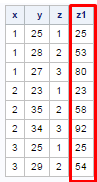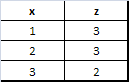# Retain Statement in SAS

This tutorial explains how to use retain statement in SAS. In SAS, it's a very easy and useful way to retain values with RETAIN statement.

Create Sample Data

The following program creates a sample data for demonstration -
data abcd;
input x y;
cards;
1 25
1 28
1 27
2 23
2 35
2 34
3 25
3 29
;
run;
Uses of RETAIN Statement

The RETAIN statement simply copies retaining values by telling the SAS not to reset the variables to missing at the beginning of each iteration of the DATA step. If you would not use retain statement then SAS would return missing at the beginning of each iteration.
The retain statement keeps the value once assigned.

Generate Serial Number

Suppose you need to generate a serial number (or row index number) with data step.
data aaa;
set abcd;
retain z 0;
z = z + 1;
run;
We can retain implicitly by using the +1 notation.
data aaa;
set abcd;
z + 1;
run;

Output DatasetOutput Data Set
Cumulative Score

Suppose you need to calculate cumulative score. In financial data, we generally need to calculate cumulative score year to date.
data aaa;
set abcd;
retain z 0;
z = z + y;
run;Output Data Set
Generate Serial Number by Group

Suppose you have a grouping variable say "region" and you need to generate a row index number by region.
proc sort data = abcd;
by x;
run;

data aaa;
set abcd;
retain z;
if first.x then z = 1;
else z = z + 1;
by x;
run;SAS : Retain Statement

Cumulative Score by Group

Suppose you need to calculate cumulative sale by product categories.
data aaa1;
set aaa;
retain z1;
if first.x then z1 = y;
else z1 = z1 + y;
by x;
run;SAS : Cumulative Score
z1 constitutes cumulative values of variable y by grouping variable x.

Number of Unique Observations

The number of unique rows by a group can easily be calculated with PROC FREQ and PROC MEANS. The following program explains how we can calculate number of observations in a categorical variable with Data Step.
data aaa2;
set abcd (drop = y);
retain z;
if first.x then z = 1;
else z = z + 1;
by x;
if last.x then output;
run;Unique Count

Suppose you have more than 1 grouping variable
data temp;
input ID ID1 Score;
cards;
1 1 25
1 1 26
1 2 27
1 2 29
2 1 28
2 1 29
2 2 31
;
run;
data temp2;
set temp;
by ID ID1;
if first.ID or first.ID1 then N = 1;
else N+1;
proc print;
run;
When you have more than 1 grouping variable, we can use multiple FIRST. statements with OR operator to generate serial numbers.Share
Related PostsDeepanshu founded ListenData with a simple objective - Make analytics easy to understand and follow. He has over 10 years of experience in data science. During his tenure, he has worked with global clients in various domains like Banking, Insurance, Private Equity, Telecom and Human Resource.

11 Responses to "Retain Statement in SAS"
1.Hi,
Thank you for making it so easy to understand took me just 1 min to understand the coding...great work brother.
Also can you please help me how can i prepare better for my base sas exam as it due in dec.

1.2.Can you explain...how to find out the middlest observation in a data set?????

2.Hi,
thank you for your clear information.
how can we find out cumulative totals for salary on each by group of gender?

1.Check the code below -

data abcd;
input Gender\$ Salary;
cards;
M 25
M 26
M 27
F 23
F 24
F 25
;
run;

proc sort data = abcd;
by gender;
run;

data temp;
set abcd;
by gender;
retain tot ;
if first.gender then tot = salary;
else tot = tot + salary;
if last.gender;
drop salary;
run;

Hope it helps!

2.data ds;
set sashelp.class;
run;
proc sort data=ds;
by sex;
run;
data ds;
set ds;
by sex;
retain cum_height 0 cum_weight 0;
if first.sex then cum_height=height;
else cum_height=cum_height+height;
if first.sex then cum_weight=weight;
else cum_weight=cum_weight+weight;
run;

3.This is not what i want.

1.Please post your sample data with desired output. It's hard to guess the requirement. Thanks!

4.Hi Deepanshu,

Thanks!!

5.Can you tell me
how to create data lines in missing values?

6.seems the sort is not a must.

Next → ← Prev
Love this post? Support Us!